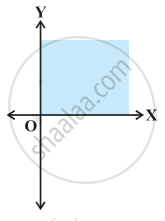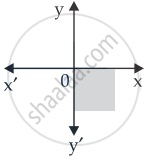# Solution set of x ≥ 0 and y ≤ 0 is - Mathematics

MCQ
True or False

Solution set of x ≥ 0 and y ≤ 0 is• True

• False

#### Solution

This statement is False.

Explanation:

Solution set of x ≥ 0 and y ≤ 0 isConcept: Solution of System of Linear Inequalities in Two Variables
Is there an error in this question or solution?

#### APPEARS IN

NCERT Mathematics Exemplar Class 11
Chapter 6 Linear Inequalities
Exercise | Q 31.(xiii) | Page 112

Share